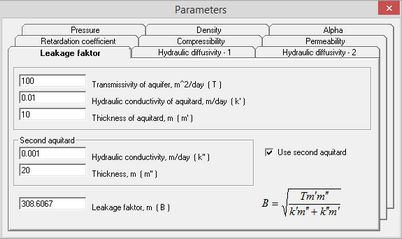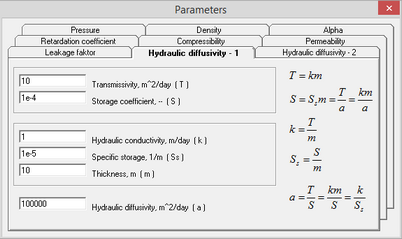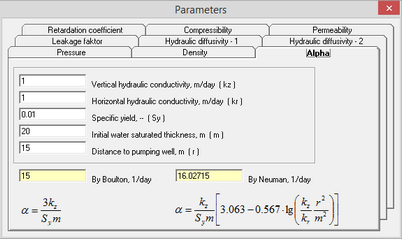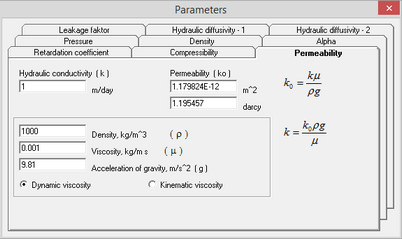﻿ Help > Parameters

# Parameters

The dialog window "Parameters" is a built-in calculator for parameters that are commonly used in hydrogeology. These parameters include leakage factor, permeability, compressibility etc.

The dialog window can be opened by selecting menu "Help > Parameters..."Tab examples of the dialog window "Parameters".

All tabs in the dialog window show the formulas, that were used to calculate the parameters.

Explanatory table

 Tab "Leakage factor" Calculates the leakage factor when one or two leaky layers are present. If a value is entered in the "Leakage factor" field, the hydraulic conductivity of the leaky layer is calculated. When two leaky layers are present, the hydraulic conductivity is calculated for the lowest layer Tab "Hydraulic diffusivity for a confined aquifer" Calculates the hydraulic diffusivity for a confined aquifer. If a value is entered in the "Hydraulic diffusivity" field, the storage coefficient is calculated. Other parameters, such as hydraulic conductivity and transmissivity, are recalculated automatically if one of the fields is filled in Tab "Hydraulic diffusivity for an confined aquifer" Calculates the hydraulic diffusivity for an unconfined aquifer based on hydraulic conductivity, thickness and specific yield. If a value is entered in the "Hydraulic diffusivity" field, the specific yield is calculated Tab "Retardation coefficient" Calculates the retardation coefficient of the river bed. This parameter is inverse to the river bed conductance parameter Tab "Compressibility" Converts compressibility to specific storage and specific storage to compressibility with known porosity and water density Tab "Permeability" Converts hydraulic conductivity to permeability and permeability to hydraulic conductivity with known water density and viscosity Tab "Pressure" Converts pressure to hydraulic head and hydraulic head to pressure Tab "Density" Calculates water density based on its total dissolved solids value Tab "Alpha" Calculates the empirical parameter alpha, required for inputs in drawdown solutions for unconfined aquifers. The parameter is calculated by two methods: the Bolton method (the most common) and the Neuman method